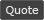WoWInterface - View Single Post - Changing Text Formatting
 Thread: Changing Text Formatting View Single Post
 05-06-17, 08:41 AM #1 cokedrivers A Rage Talon Dragon Guard Join Date: Aug 2009 Posts: 316 Changing Text Formatting As the title says I want to change some text, I have 2 codes but they do not match as things are going on. I would like tyo use the Frist Code for everything. First Code (This code is being used for my Nameplates): Code: ```local FormatValue = function(number) if number < 1e3 then return floor(number) elseif number >= 1e12 then return string.format("%.3fT", number/1e12) elseif number >= 1e9 then return string.format("%.3fB", number/1e9) elseif number >= 1e6 then return string.format("%.2fM", number/1e6) elseif number >= 1e3 then return string.format("%.1fK", number/1e3) end end``` Second Code (This code is being used for my UnitFrames): Code: ``` local shorts = { { 1e10, 1e9, "%.0fB" }, -- 10b+ as 12B { 1e9, 1e9, "%.1fB" }, -- 1b+ as 8.3B { 1e7, 1e6, "%.0fM" }, -- 10m+ as 14M { 1e6, 1e6, "%.1fM" }, -- 1m+ as 7.4M { 1e5, 1e3, "%.0fK" }, -- 100k+ as 840K { 1e3, 1e3, "%.1fK" }, -- 1k+ as 2.5K { 0, 1, "%d" }, -- < 1k as 974 } for i = 1, #shorts do shorts[i] = shorts[i] .. " (%.0f%%)" end hooksecurefunc("TextStatusBar_UpdateTextStringWithValues", function(statusBar, textString, value, valueMin, valueMax) if value == 0 then return textString:SetText("") end local style = GetCVar("statusTextDisplay") if style == "PERCENT" then return textString:SetFormattedText("%.0f%%", value / valueMax * 100) end for i = 1, #shorts do local t = shorts[i] if value >= t then if style == "BOTH" then return textString:SetFormattedText(t, value / t, value / valueMax * 100) else if value < valueMax then for j = 1, #shorts do local v = shorts[j] if valueMax >= v then return textString:SetFormattedText(t .. " / " .. v, value / t, valueMax / v) end end end return textString:SetFormattedText(t, value / t) end end end end)``` Hopefully someone can help me with the code. Thanks Coke Last edited by cokedrivers : 05-06-17 at 09:10 AM.